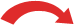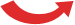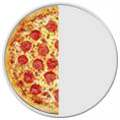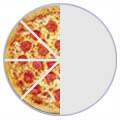## Equivalent Fractions

Tilly (11) and James (8) have been learning about equivalent fractions.

This is them in action!Equivalent Fractions have the same value, even though they may look different.

These fractions are really the same:

1     2     4
/  =  /  =  /
2     4     8

Why are they the same? Because when you multiply or divide both the top and bottom by the same number, the fraction keeps it's value.

The rule to remember is:

### What you do to the top of the fraction you must also do to the bottom of the fraction !

So, here is why those fractions are really the same:

 × 2 × 21 = 2 = 4 / / / 2 4 8× 2 × 2
And visually it looks like this:

 1/2 2/4 4/8==script>